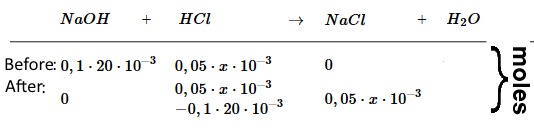Search:

# Evolution of the pH during the titration of a strong base by a strong acidThereafter we call $x$ the volume $(mL)$ of $HCl$ already added.

### Initial concentration of the base

$c_B$ = $\frac{c_{Α}V_{Α}}{V_B}$ = $\frac{0.05\cdot 0.040}{0.020}$ = $0.10 \frac{mol}{L}$

1.

### $pH$ at the beginning of the titration  $(x=0)$

The strong base $NaOH$ is present alone. its concentration is $c_B$ $=$ $0.10 \;M$ $pH$ $=$ $14$ $+$ $logc_B$ = $13$

2.

### $pH$ before the equivalence point  $(x\;\lt\;40)$

(For simplicity. we consider the substances first as if they were not dissociated)$c_{NaOH}$ = $\frac{n_{NaOH}}{(V_B+x)10^{-3}}$ = $\frac{0.1\cdot20\cdot10^{-3}-0.05\cdot x\cdot10^{-3}}{(20+x)10^{-3}}$ = $\frac{2-0.05\cdot x}{20+x}$ $pH$ $=$ $14$ $+$ $log( \frac{2-0.05\cdot x}{20+x})$

3.

### $pH$ at the equivalence point  $(x\;=\;40)$

$HCl$ and $NaOH$ reacted completely. Remains a neutral $NaCl$ solution: $pH = 7$

4.

### $pH$ after the equivalence point  $(x\;\gt\;40)$$c_{HCl}$ = $\frac{n_{HCl}}{(V_B+x)10^{-3}}$ = $\frac{0.05\cdot x\cdot10^{-3}-0.1\cdot20\cdot10^{-3}}{(20+x)10^{-3}}$ = $\frac{0.05\cdot x-2}{20+x}$ $pH$ $=$ $-log( \frac{0.05\cdot x-2}{20+x})$

→   Here you find simulations of such titrations